MathScore EduFighter is one of the best math games on the Internet today. You can start playing for free!

## Mean, Median, Mode - Sample Math Practice Problems

The math problems below can be generated by MathScore.com, a math practice program for schools and individual families. References to complexity and mode refer to the overall difficulty of the problems as they appear in the main program. In the main program, all problems are automatically graded and the difficulty adapts dynamically based on performance. Answers to these sample questions appear at the bottom of the page. This page does not grade your responses.

See some of our other supported math practice problems.

### Complexity=1, Mode=simple

Find the mean, median, mode, and range. If there is more than one mode, answer in the form "5 8" where the modes are 5 and 8. If there is no mode, leave it blank.

 1.   4, 4 mean median mode range 2.   7, 5 mean median mode range

### Complexity=3, Mode=simple

Find the mean, median, mode, and range. If there is more than one mode, answer in the form "5 8" where the modes are 5 and 8. If there is no mode, leave it blank.

 1.   1, 0, 9, 7, 3 mean median mode range 2.   2, 6, 2, 6 mean median mode range

### Complexity=1, Mode=graph

Find the mean, median, mode, and range. If there is more than one mode, answer in the form "5 8" where the modes are 5 and 8. If there is no mode, leave it blank.

 1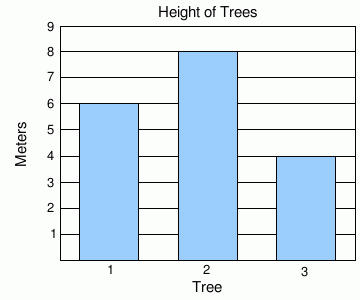mean median mode range 2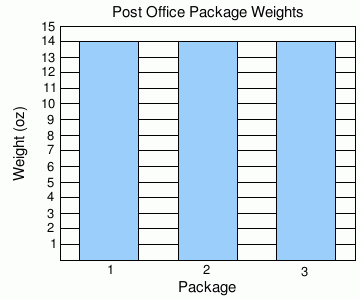mean median mode range

### Complexity=2, Mode=graph

Find the mean, median, mode, and range. If there is more than one mode, answer in the form "5 8" where the modes are 5 and 8. If there is no mode, leave it blank.

 1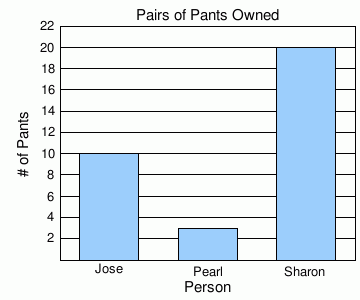mean median mode range 2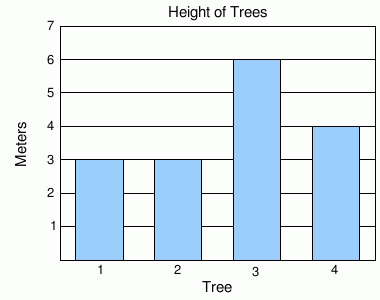mean median mode range

### Complexity=3, Mode=graph

Find the mean, median, mode, and range. If there is more than one mode, answer in the form "5 8" where the modes are 5 and 8. If there is no mode, leave it blank.

 1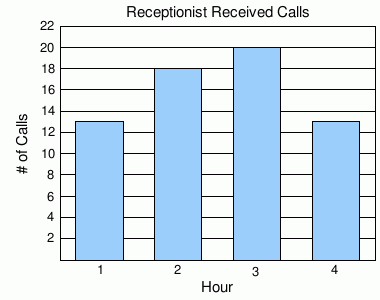mean median mode range 2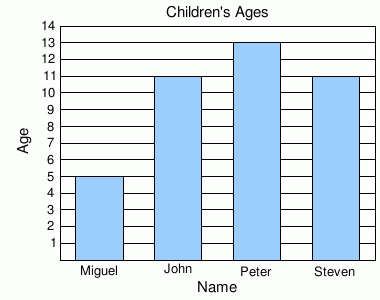mean median mode range

### Complexity=5, Mode=graph

Find the mean, median, mode, and range. If there is more than one mode, answer in the form "5 8" where the modes are 5 and 8. If there is no mode, leave it blank.

 1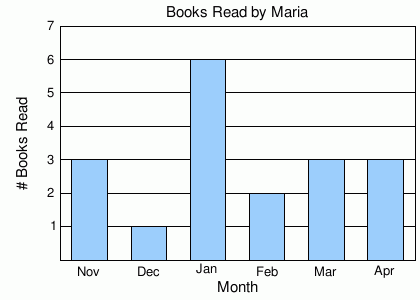mean median mode range 2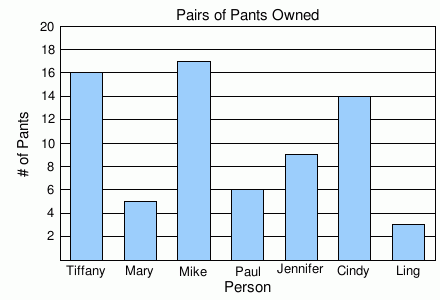mean median mode range

### Complexity=2, Mode=table

Find the mean, median, mode, and range. If there is more than one mode, answer in the form "5 8" where the modes are 5 and 8. If there is no mode, leave it blank.

1.

Temperature of Summer Day
SatSunMonTue
88918588

mean
median
mode
range
2.

Distance Driven on Vacation
Day 1Day 2Day 3Day 4
16019320291

mean
median
mode
range

### Complexity=3, Mode=table

Find the mean, median, mode, and range. If there is more than one mode, answer in the form "5 8" where the modes are 5 and 8. If there is no mode, leave it blank.

1.

Weight in Kilograms
ClaudiaJeffGraceJuanita
81686352

mean
median
mode
range
2.

Music CDs Owned
AntonioGregChristineIsabellaMatt
3622264135

mean
median
mode
range

### Complexity=5, Mode=table

Find the mean, median, mode, and range. If there is more than one mode, answer in the form "5 8" where the modes are 5 and 8. If there is no mode, leave it blank.

1.

Test Scores per Test
Test Number 1Test Number 2Test Number 3Test Number 4Test Number 5Test Number 6
866762826477

mean
median
mode
range
2.

Weight in Pounds
LizHermioneSamJustinJackJasmineMichi
10711611417096105118

mean
median
mode
range

### Complexity=1, Mode=simple

Find the mean, median, mode, and range. If there is more than one mode, answer in the form "5 8" where the modes are 5 and 8. If there is no mode, leave it blank.

14, 4
mean
median
mode
range
Solution
To get the mean:
The sum is 8
Divide this by 2 to get the mean.
The mean is 4

To get the median:
The median is the average of the two middle numbers. (4 + 4) / 2 = 4

To get the mode:
4 is the mode because it occurs the most number of times

To get the range:
The minimum number is 4. The maximum number is 4. The range is 4 - 4 = 0.

27, 5
mean
median
mode
range
Solution
To get the mean:
The sum is 12
Divide this by 2 to get the mean.
The mean is 6

To get the median:
The median is the average of the two middle numbers. (5 + 7) / 2 = 6

To get the mode:
There is no mode because there are no duplicate numbers.

To get the range:
The minimum number is 5. The maximum number is 7. The range is 7 - 5 = 2.

### Complexity=3, Mode=simple

Find the mean, median, mode, and range. If there is more than one mode, answer in the form "5 8" where the modes are 5 and 8. If there is no mode, leave it blank.

11, 0, 9, 7, 3
mean
median
mode
range
Solution
To get the mean:
The sum is 20
Divide this by 5 to get the mean.
The mean is 4

To get the median:
The middle number is 3,

To get the mode:
There is no mode because there are no duplicate numbers.

To get the range:
The minimum number is 0. The maximum number is 9. The range is 9 - 0 = 9.

22, 6, 2, 6
mean
median
mode
range
Solution
To get the mean:
The sum is 16
Divide this by 4 to get the mean.
The mean is 4

To get the median:
The median is the average of the two middle numbers. (2 + 6) / 2 = 4

To get the mode:
Multiple numbers occurred the most frequently, (2 times), so we have multiple modes: 2 6

To get the range:
The minimum number is 2. The maximum number is 6. The range is 6 - 2 = 4.

### Complexity=1, Mode=graph

Find the mean, median, mode, and range. If there is more than one mode, answer in the form "5 8" where the modes are 5 and 8. If there is no mode, leave it blank.

1mean
median
mode
range
Solution
To get the mean:
The sum is 18
Divide this by 3 to get the mean.
The mean is 6

To get the median:
The middle number is 6,

To get the mode:
There is no mode because there are no duplicate numbers.

To get the range:
The minimum number is 4. The maximum number is 8. The range is 8 - 4 = 4.

2mean
median
mode
range
Solution
To get the mean:
The sum is 42
Divide this by 3 to get the mean.
The mean is 14

To get the median:
The middle number is 14,

To get the mode:
14 is the mode because it occurs the most number of times

To get the range:
The minimum number is 14. The maximum number is 14. The range is 14 - 14 = 0.

### Complexity=2, Mode=graph

Find the mean, median, mode, and range. If there is more than one mode, answer in the form "5 8" where the modes are 5 and 8. If there is no mode, leave it blank.

1mean
median
mode
range
Solution
To get the mean:
The sum is 33
Divide this by 3 to get the mean.
The mean is 11

To get the median:
The middle number is 10,

To get the mode:
There is no mode because there are no duplicate numbers.

To get the range:
The minimum number is 3. The maximum number is 20. The range is 20 - 3 = 17.

2mean
median
mode
range
Solution
To get the mean:
The sum is 16
Divide this by 4 to get the mean.
The mean is 4

To get the median:
The median is the average of the two middle numbers. (3 + 4) / 2 = 3.5

To get the mode:
3 is the mode because it occurs the most number of times

To get the range:
The minimum number is 3. The maximum number is 6. The range is 6 - 3 = 3.

### Complexity=3, Mode=graph

Find the mean, median, mode, and range. If there is more than one mode, answer in the form "5 8" where the modes are 5 and 8. If there is no mode, leave it blank.

1mean
median
mode
range
Solution
To get the mean:
The sum is 64
Divide this by 4 to get the mean.
The mean is 16

To get the median:
The median is the average of the two middle numbers. (13 + 18) / 2 = 15.5

To get the mode:
13 is the mode because it occurs the most number of times

To get the range:
The minimum number is 13. The maximum number is 20. The range is 20 - 13 = 7.

2mean
median
mode
range
Solution
To get the mean:
The sum is 40
Divide this by 4 to get the mean.
The mean is 10

To get the median:
The median is the average of the two middle numbers. (11 + 11) / 2 = 11

To get the mode:
11 is the mode because it occurs the most number of times

To get the range:
The minimum number is 5. The maximum number is 13. The range is 13 - 5 = 8.

### Complexity=5, Mode=graph

Find the mean, median, mode, and range. If there is more than one mode, answer in the form "5 8" where the modes are 5 and 8. If there is no mode, leave it blank.

1mean
median
mode
range
Solution
To get the mean:
The sum is 18
Divide this by 6 to get the mean.
The mean is 3

To get the median:
The median is the average of the two middle numbers. (3 + 3) / 2 = 3

To get the mode:
3 is the mode because it occurs the most number of times

To get the range:
The minimum number is 1. The maximum number is 6. The range is 6 - 1 = 5.

2mean
median
mode
range
Solution
To get the mean:
The sum is 70
Divide this by 7 to get the mean.
The mean is 10

To get the median:
The middle number is 9,

To get the mode:
There is no mode because there are no duplicate numbers.

To get the range:
The minimum number is 3. The maximum number is 17. The range is 17 - 3 = 14.

### Complexity=2, Mode=table

Find the mean, median, mode, and range. If there is more than one mode, answer in the form "5 8" where the modes are 5 and 8. If there is no mode, leave it blank.

1
Temperature of Summer Day
SatSunMonTue
88918588

mean
median
mode
range
Solution
To get the mean:
The sum is 352
Divide this by 4 to get the mean.
The mean is 88

To get the median:
The median is the average of the two middle numbers. (88 + 88) / 2 = 88

To get the mode:
88 is the mode because it occurs the most number of times

To get the range:
The minimum number is 85. The maximum number is 91. The range is 91 - 85 = 6.

2
Distance Driven on Vacation
Day 1Day 2Day 3Day 4
16019320291

mean
median
mode
range
Solution
To get the mean:
The sum is 664
Divide this by 4 to get the mean.
The mean is 166

To get the median:
The median is the average of the two middle numbers. (160 + 193) / 2 = 176.5

To get the mode:
There is no mode because there are no duplicate numbers.

To get the range:
The minimum number is 20. The maximum number is 291. The range is 291 - 20 = 271.

### Complexity=3, Mode=table

Find the mean, median, mode, and range. If there is more than one mode, answer in the form "5 8" where the modes are 5 and 8. If there is no mode, leave it blank.

1
Weight in Kilograms
ClaudiaJeffGraceJuanita
81686352

mean
median
mode
range
Solution
To get the mean:
The sum is 264
Divide this by 4 to get the mean.
The mean is 66

To get the median:
The median is the average of the two middle numbers. (63 + 68) / 2 = 65.5

To get the mode:
There is no mode because there are no duplicate numbers.

To get the range:
The minimum number is 52. The maximum number is 81. The range is 81 - 52 = 29.

2
Music CDs Owned
AntonioGregChristineIsabellaMatt
3622264135

mean
median
mode
range
Solution
To get the mean:
The sum is 160
Divide this by 5 to get the mean.
The mean is 32

To get the median:
The middle number is 35,

To get the mode:
There is no mode because there are no duplicate numbers.

To get the range:
The minimum number is 22. The maximum number is 41. The range is 41 - 22 = 19.

### Complexity=5, Mode=table

Find the mean, median, mode, and range. If there is more than one mode, answer in the form "5 8" where the modes are 5 and 8. If there is no mode, leave it blank.

1
Test Scores per Test
Test Number 1Test Number 2Test Number 3Test Number 4Test Number 5Test Number 6
866762826477

mean
median
mode
range
Solution
To get the mean:
The sum is 438
Divide this by 6 to get the mean.
The mean is 73

To get the median:
The median is the average of the two middle numbers. (67 + 77) / 2 = 72

To get the mode:
There is no mode because there are no duplicate numbers.

To get the range:
The minimum number is 62. The maximum number is 86. The range is 86 - 62 = 24.

2
Weight in Pounds
LizHermioneSamJustinJackJasmineMichi
10711611417096105118

mean
median
mode
range
Solution
To get the mean:
The sum is 826
Divide this by 7 to get the mean.
The mean is 118

To get the median:
The middle number is 114,

To get the mode:
There is no mode because there are no duplicate numbers.

To get the range:
The minimum number is 96. The maximum number is 170. The range is 170 - 96 = 74.